### We can't stand without you, GRAVITATION.

Let's find out the mystery of gravitation with Newton's eyes!

### Do you think this hammock will fall?

Let's find out what is static equilibrium to discover the answer.

### I didn't listen the song but I saw the record had been rotated.

If you are interested to discover what is rotation instead of the song, please click on me and you will get what you want.

### Circular motion, it's not simple as a circle.

Let's understand the circular motion of the roller coaster and the force acting on it.

### Are you working?!?

Let's find out how much work is done by you and how much energy and power dissipated during work.

## Sunday, 18 March 2012

### Gravitation

Newton ‘s law of gravitation states that the force of attraction between particles is directly proportional to their mass and inversely proportional to the square of distance apart.

Where G is the universal gravitational constant,

7.2 GRAVITATIONAL FIELD STRENGTH
A gravitational field is a region where gravitational force acts on massive bodies. Eg . gravitaional field of the Earth.
The gravitational field strength tells us how strong a gravitational field is. The gravitational field strength of the Earth near its surface is 9.81m / s2.
The gravitational field strength , E at a point is the force of gravity per unit mass exerted on a mass placed.

The variation of the acceleration due to gravity g’ with distance r from the centre of the Earth is illustrated by the graph above.

7.3 Gravitational Potential
The strength of the gravitational force at a point in a gravitational field is described by the gravitational field strength E or g is a vector quantity.
Another quantity associated with the point in the gravitational field is the gravitational potential. It is a scalar quantity.
The gravitational potential V at a point P in a gravitational field is defined as the work done per unit mass to bring a body from infinity to P. The unit for gravitational potential is Jkgˉ¹.
The gravitational potential energy U of a body at a point P in a gravitational field is defined as the work done to bring the body from infinity to P. The unit for gravitational potential energy is J.
Hence the gravitational potential energy U of a body of mass m at a point where the gravitational potential,is given by
U = mV

On the surface on the Earth, r = R
Gravitational potential, V = -
Gravitational potential energy, U = -
The graph illustrates the variation of the gravitational potential V with distance r from the centre of the Earth.

7.4 RELATIONSHIP BETWEEN g AND G
-G is the Universal Gravitational Constant.
- It is a scalar quantity with dimension

- It is a vector quantity with dimension

R = constant radius of earth
G = universal gravitational constant
M = mass of Earth

7.5 Satellite Motion in Circular Orbits

Satellite is a body that revolves round a planet. Satellites can be categorized as natural satellites or man-made satellites. The moon, the planets and comets are examples of natural satellites. Examples of man-made satellites are Sputnik I , Measat I ,II and III which are   communication satellites. In order to launch satellite into orbit , rockets are used. When rocket that carries the satellite reaches the required height , the satellite is launched into circular orbit with a certain velocity v that is tangential to intended orbit.

### Frictional Forces

Friction is the force opposing the motion of one body sliding or rolling over the surface of second object. Several aspects of friction are important at low velocities:

• The direction of the force of friction is opposite the direction of motion.
• The frictional force is proportional to the perpendicular (normal) force between the two surfaces in contact.
• The frictional force is nearly independent of the area of contact between the two objects.
• The magnitude of the frictional force depends on the materials composing the two objects in contact.

Static friction is the force of friction when there is no relative motion between two objects in contact, such as a block sitting on an inclined plane.
The magnitude of the frictional force is Fs ≤ μs N, where N is the magnitude of the normal force, and the coefficient of friction (μs) is the dimensionless proportionality constant.
The coefficient of friction may be approximately .2 for normal lubricated surfaces and close to 1 for glass sliding on glass. This equation sets the upper limit for the static frictional force. If a greater external force is applied, the situation will no longer be static, and the object will begin to move.

Kinetic friction is the force of friction when there is relative motion between two objects in contact.
The magnitude of the friction force in this case is F k ≤ μk N, where N is the magnitude of the normal force and μ k is the coefficient of kinetic friction. Note that μ k is not strictly a constant, but this empirical rule is a good approximation for finding frictional forces. Values given for the coefficients of static and kinetic friction do vary with speed and surface conditions so that it is not necessarily true that static friction exceeds sliding friction.

The following problem highlights the differences between static and kinetic friction.

Example 6: A block sits on an inclined plane. What is the maximum angle that which the block remains at rest? First, draw the free-body diagrams and then write out the force equation for each direction of the coordinate system (see Figure 6 ).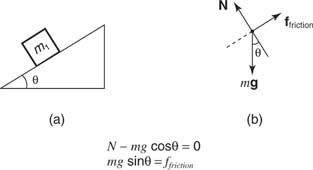Figure 6 A block on an inclined plane, with friction.

Suppose the surface is tilted to θ, at which the block just begins to move. Then, the force down the plane must be equal to the maximum force of static friction; thus f friction = μs N. Therefore, f friction = mg sin θ = μs N = μs ( mg cos θ) and solving for the coefficient of friction:

Solution: μs = tan θ

At a greater angle of tilt, the object accelerates down the surface, and the force of friction is f k = μ k N.

Example 7: If the surfaces in Figures 4 and 5 were not frictionless, the frictional force parallel to the surface and opposite the direction of motion must be included in the analysis. Pulling a block along a horizontal surface at a constant speed (zero acceleration) is an example of a problem involving friction, and such a block is analyzed in Figure 7 .Figure 7 Pulling a block on a plane with friction.

In the x direction, T cos θ − f = 0, where f is the friction force and T is the tension in the rope. In the y direction, N + T sin θ − mg = 0; also, f = μk N.

Solve the y direction equation for N, substitute the expression into the friction force equation, and then substitute friction into the first equation to obtain the following: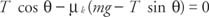Solution: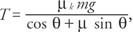solving for T.

### Equilibrium of a Rigid Body

The Moment of a Force

The moment M (turning effect) of a force about a point O is the product of the magnitude of the force (F) and the perp. distance (x)to the point of application.By convention, anti-clockwise moments are positive.

The Principle of Moments

For a rigid body acted upon by a system of coplanar forces, equilibrium is achieved when:

i)  the vector sum of the coplanar forces = 0
ii) there is no net turning effect produced by the forces
(the sum of clockwise & anti-clockwise moments = 0)

Parallel forces acting on a beam

When attempting problems concerning a balance points or fulcrum, remember that there is always an upward force acting.

Example #1   fulcrum near centreConsider two forces F1 and F2 acting vertically downwards at either end of a beam of negligible mass.
When the beam is balanced at its fulcrum O(i.e. horizontal), the sum of the downward forces equals the sum of the upward forces.
If the reaction force at the fulcrum is R,Example #2   fulcrum at one endConsider two forces F1 and F2 acting on a beam of negligible mass. One force acts vertically downwards near the centre, while the other acts vertically upwards at the end.
When the beam is balanced, the sum of the downward forces equals the sum of the upward forces.
If the reaction force at the fulcrum is R,Example #3    a typical problemA beam of negligible weight is horizontal in equilibrium, with forces acting upon it, as shown in the diagram.
Calculate the value of the weights R and W.CouplesThe turning effect of two equal and opposite parallel forces acting about a point equals the product of one force(F) and the perpendicular distance between the forces(d).
couple = F x d

### Equilibrium of Particles

Equilibrium equations for a particle:
A particle is in equilibrium if the resultant of ALL forces acting on the particle is equal to zero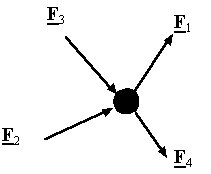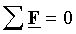Equilibrium equations in component form:
In a rectangular coordinate system the equilibrium equations can be represented by three scalar equations: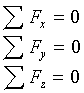Triangle of Forces
When 3 coplanar forces acting at a point are in equilibrium, they can be represented in magnitude and direction by the adjacent sides of a triangle taken in order.Example
Using the results from the previous example, the three forces acting on the 2 kg mass can be represented by a scale diagram.Our starting point is the 20N force acting downwards. One force acts at 45 deg. to this line and the other at 60 deg. So to find the magnitude of the two forces, draw lines at these angles at each end of the 20N force. Where the lines cross gives a vertex of the triangle. Measuring the lengths of the lines from this to the ends of the 20N force line will give the magnitudes of the required forces.

Polygon of Forces
For equilibrium, forces are represented in magnitude and direct to form a polygon shape.
If a number of forces are acting at a point, then the missing side in the polygon represents the resultant force. Note the arrow direction on this force is in the opposite direction to the rest.